Next: Higher Order Methods Up: Numerical Solution of Initial Previous: Numerical Solution of Initial

## Forward and Backward Euler Methods

Let's denote the time at the nth time-step by tn and the computed solution at the nth time-step by yn, i.e.,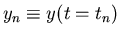. The step size h (assumed to be constant for the sake of simplicity) is then given by h = tn - tn-1. Given (tn, yn), the forward Euler method (FE) computes yn+1 as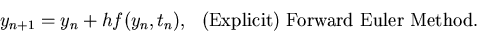(6)

The forward Euler method is based on a truncated Taylor series expansion, i.e., if we expand y in the neighborhood of t=tn, we get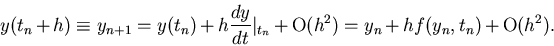(7)

From (8), it is evident that an error is induced at every time-step due to the truncation of the Taylor series, this is referred to as the local truncation error (LTE) of the method. For the forward Euler method, the LTE is O(h2). Hence, the method is referred to as a first order technique. In general, a method with O(hk+1) LTE is said to be of kth order. Evidently, higher order techniques provide lower LTE for the same step size. The truncation error is different from the global error gn, which is defined as the absolute value of the difference between the true solution and the computed solution, i.e., gn = |ye(tn)-yn|. In most cases, we do not know the exact solution and hence the global error is not possible to be evaluated. However, if we neglect roundoff errors, it is reasonable to assume that the global error at the nth time step is n times the LTE, since n is proportional to 1/h, gn should be proportional to LTE/h. This implies that for a kth order method, the global error scales as hk.

A convergent numerical method is the one where the numerically computed solution approaches the exact solution as the step size approaches 0. Once again, if the true solution is not known a priori, we can choose, depending on the precision required, the solution obtained with a sufficiently small time step as the 'exact' solution to study the convergence characteristics.

Another important observation regarding the forward Euler method is that it is an explicit method, i.e., yn+1 is given explicitly in terms of known quantities such as yn and f(yn,tn). Explicit methods are very easy to implement, however, the drawback arises from the limitations on the time step size to ensure numerical stability. In order to see this better, let's examine a linear IVP, given by dy/dt = -ay, y(0)=1 with a>0. As we know, the exact solution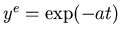, which is a stable and a very smooth solution with ye(0) = 1 and. Now, what is the discrete equation obtained by applying the forward Euler method to this IVP? Using Eq. 7, we get

 yn+1 = yn -ah yn = (1-ah) yn = (1-ah)2 yn-1 = ... = (1-ah)n y1 = (1-ah)n+1 y0. (8)

Eq. 9 implies that in order to prevent the amplification of the errors in the iteration process, we require |1-ah| < 1 or for stability of the forward Euler method, we should have h<2/a.

These results can be better perceived from Figures 1 and 2. The test problem is the IVP given by dy/dt = -10y, y(0)=1 with the exact solution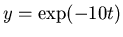. The stability criterion for the forward Euler method requires the step size h to be less than 0.2. In Figure 1, we have shown the computed solution for h=0.001, 0.01 and 0.05 along with the exact solution1. As seen from there, the method is numerically stable for these values of h and becomes more accurate as h decreases. However, based on the stability analysis given above, the forward Euler method is stable only for h < 0.2 for our test problem. The numerical instability which occurs for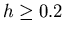is shown in Figure 2. For h =0.2, the instability is oscillatory between, whereas for h>0.2, the amplitude of the oscillation grows in time without bound, leading to an explosive numerical instability.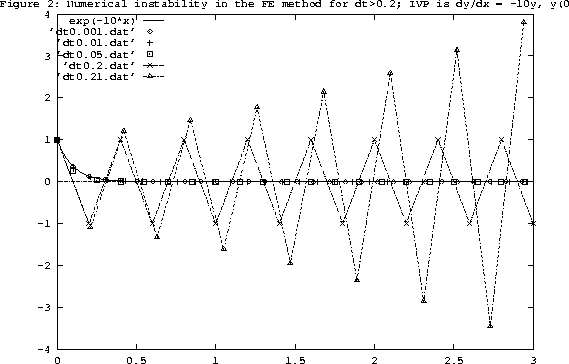The convergence of the solution can be analyzed quantitatively. Let's look at the global error gn = |ye(tn) - y(tn)| for our test problem at t=1. We know that the local truncation error (LTE) at any given step for the Euler method scales with h2. Hence, the global error gn is expected to scale with nh2. However, for the integration within a fixed time interval, n is proportional to 1/h. So the global error gn at the nth Euler step is proportional to h. This result is confirmed by the computational results presented in Figure 3, where the global error at t=1 is plotted against the time step size h.The conditional stability, i.e., the existence of a critical time step size beyond which numerical instabilities manifest, is typical of explicit methods such as the forward Euler technique. Implicit methods can be used to replace explicit ones in cases where the stability requirements of the latter impose stringent conditions on the time step size. However, implicit methods are more expensive to be implemented for non-linear problems since yn+1 is given only in terms of an implicit equation. The implicit analogue of the explicit FE method is the backward Euler (BE) method. This is based on the following Taylor series expansion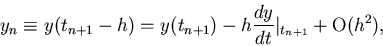(9)

which gives(10)

Once again, note that in Eq. 11, f(yn+1,tn+1) is not known, hence it gives us an implicit equation for the computation of yn+1 (Compare Eqs. 7 and 11). For instance, let. This means that to obtain yn+1, we need to solve the non-linear equationat any given time step n. A suitable root finding technique such as the Newton-Raphson method can be used for this purpose. This is evidently much more time consuming than the explicit FE method where, for the problem above, we have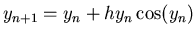.

Well, why do we resort to implicit methods despite their high computational cost? The reason is that implicit techniques are stable. Let's examine this for the same linear test problem we considered in the context of the FE method: dy/dt = -10 y, y(0) = 1. In the case of linear problems, using BE is as easy as using FE, applying Eq. 11, we have(11)

which gives a numerical scheme stable for all h>0. In Figure 4, I have plotted the solutions computed using the BE method for h=0.001, 0.01, 0.1, 0.2 and 0.5 along with the exact solution. Note that there is no numerical instability in this case. The accuracy of the computed solution deteriorates as h is increased, and we expect the global error to scale linearly with h.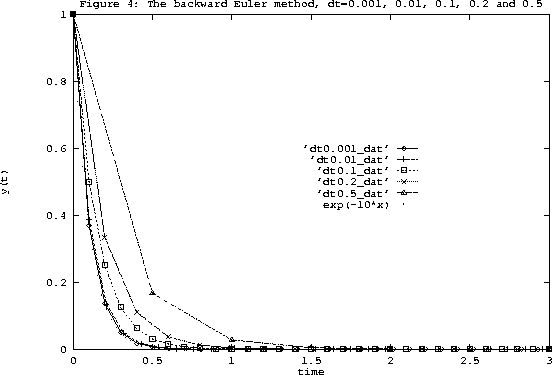Next: Higher Order Methods Up: Numerical Solution of Initial Previous: Numerical Solution of Initial
Michael Zeltkevic
1998-04-15